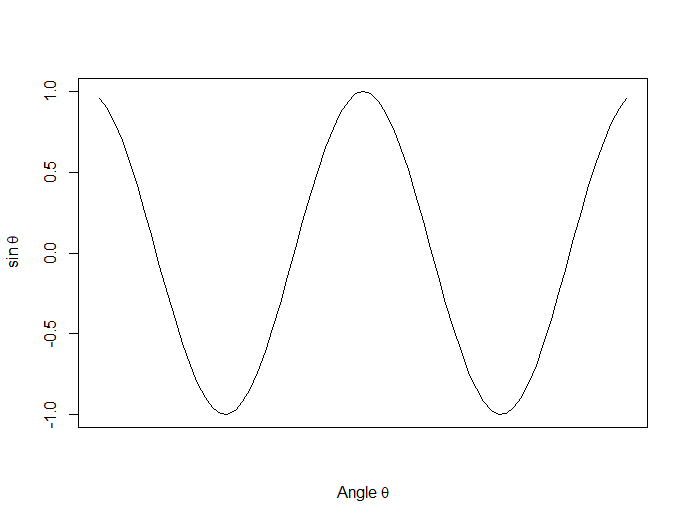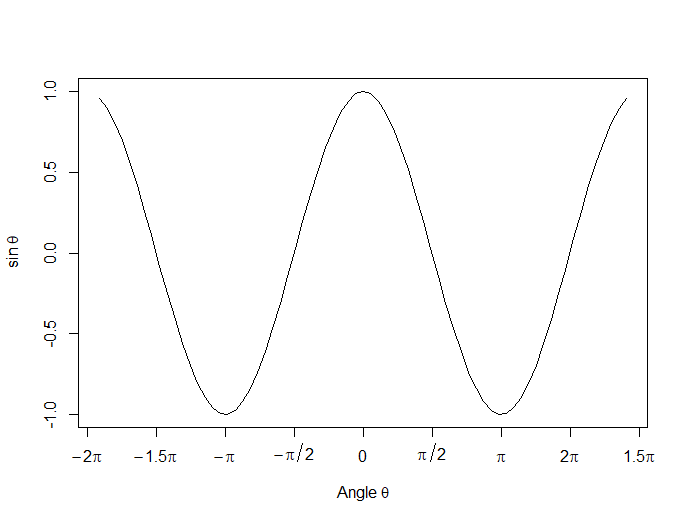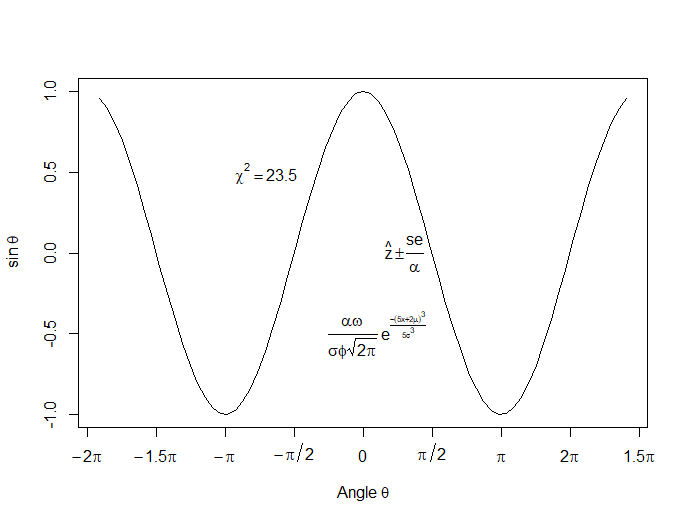# R

### R Graphics: Plotting in Color with qplot

January 12th, 2015 byIn this lesson, let’s see how to use qplot to map symbol colour to a categorical variable.

Copy in the following data set (a medical data set relating to patients in a randomised controlled trial):

### Doing Scatterplots in R

January 7th, 2015 byIn this lesson, we see how to use qplot to create a simple scatterplot.

The qplot (quick plot) system is a subset of the ggplot2 (grammar of graphics) package which you can use to create nice graphs. It is great for creating graphs of categorical data, because you can map symbol colour, size and shape to the levels of your categorical variable. To use qplot first install ggplot2 as follows:
(more…)

### Graphing Non-Linear Mathematical Expressions in R

December 30th, 2014 byIn this lesson, let’s see how to create mathematical expressions for your graph in R.  We’ll use an example of graphing a cosine curve, along with relevant Greek letters as the axis label, and printing the equation right on the graph.

Mathematical expressions, like sine or exponential curves on graphs are made possible through `expression(paste())` and `substitute()`.

If you need mathematical symbols as axis labels, switch off the default axes and include Greek symbols by writing them out in English. You can create fractions through the `frac()` command. Note how we obtain the plus or minus sign through the syntax: `%+-%`

Here is a nice example. Let’s create a set of 71 values from – 6 to + 6. These values are the horizontal axis values.

`x <- seq(-6, 6, len = 71)`

Now we plot a cosine function using a continuous curve (using `type="l"`) while suppressing the x axis using the syntax: `xaxt="n"`

```plot(x, cos(x),type="l",xaxt="n", xlab=expression(paste("Angle ",theta)), ylab=expression("sin "*theta)) ```
. . . where we have inserted relevant mathematical text for the axis labels using `expression(paste())`. Here is the graph so far:Now we create a horizontal axis to our own specifications, including relevant labels:

```axis(1, at = c(-2*pi, -1.5*pi, -pi, -pi/2, 0, pi/2, pi, 1.5*pi, 2*pi), lab = expression(-2*pi, -1.5*pi, -pi, -pi/2, 0, pi/2, pi, 2*pi, 1.5*pi))```Let’s put in some mathematical expressions, centered appropriately. The first argument within each `text()` function gives the value along the horizontal axis about which the text will be centered.

`text(-0.7*pi,0.5,substitute(chi^2=="23.5"))`

text(0.1*pi, -0.5, expression(paste(frac(alpha*omega, sigma*phi*sqrt(2*pi)), ” “,
e^{frac(-(5*x+2*mu)^3, 5*sigma^3)})))

text(0.3*pi,0,expression(hat(z) %+-% frac(se, alpha)))

Here is our graph, complete with mathematical expressions:That wasn’t so hard! In the next lesson we will discuss using qplot in R to create scatterplots.

About the Author: David Lillis has taught R to many researchers and statisticians. His company, Sigma Statistics and Research Limited, provides both on-line instruction and face-to-face workshops on R, and coding services in R. David holds a doctorate in applied statistics.

### R Graphics: Multiple Graphs and par(mfrow=(A,B))

December 16th, 2014 byToday we see how to set up multiple graphs on the same page. We use the syntax ` par(mfrow=(A,B))` (more…)

### R Is Not So Hard! A Tutorial, Part 18: Re-Coding Values

August 29th, 2014 byOne data manipulation task that you need to do in pretty much any data analysis is recode data.  It’s almost never the case that the data are set up exactly the way you need them for your analysis.

In R, you can re-code an entire vector or array at once. To illustrate, let’s set up a vector that has missing values.

`A <- c(3, 2, NA, 5, 3, 7, NA, NA, 5, 2, 6)`

`A`

` 3 2 NA 5 3 7 NA NA 5 2 6`

We can re-code all missing values by another number (such as zero) as follows: (more…)

### R Is Not So Hard! A Tutorial, Part 17: Testing for Existence of Particular Values

August 25th, 2014 bySometimes you need to know if your data set contains elements that meet some criterion or a particular set of criteria.

For example, a common data cleaning task is to check if you have missing data (NAs) lurking somewhere in a large data set.

Or you may need to check if you have zeroes or negative numbers, or numbers outside a given range.

In such cases, the any() and all() commands are very helpful. You can use them to interrogate R about the values in your data. (more…)